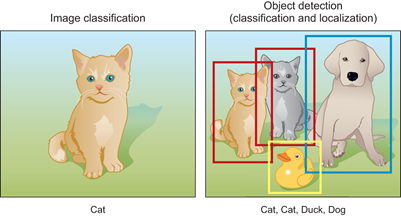# 7 Object Detection with R-CNN, SSD, and YOLO

#### “The eye sees only what the mind is prepared to comprehend.”

-- Robertson Davies

### Learning goals from this chapter:

• Understand the difference between image classification and object detection tasks
• Understand the general framework of object detection projects
• Learn how to use different object detection algorithms like R-CNN, SSD, and YOLO
• By the end of this chapter, we will have gained an understanding of how deep learning is applied to object detection, and how the different object detection models inspire and diverge from one another.

In the previous chapters, we explained how we can use deep neural networks for image classification tasks. In image classification, we assume that there is only one main target object in the image and the model’s sole focus is to identify the target category. However, in many situations, there are multiple targets in the image that we are interested in. We not only want to classify them, but also want to obtain their specific positions in the image. In computer vision, we refer to such tasks as object detection. See Figure 7.1 that explains the difference between image classification and object detection tasks.

##### Figure 7.1: Image Classification vs. Object Detection tasks. In classification tasks, the classifier outputs the class probability (cat) whereas, in object detection tasks, the detector outputs the bounding box coordinates (4 boxes in this example) and the predicted classes (2 cats + duck + dog).